# JP2013171491A - Traffic estimation system using single image - Google Patents

## Info

Publication number
JP2013171491A
JP2013171491A JP2012035956A JP2012035956A JP2013171491A JP 2013171491 A JP2013171491 A JP 2013171491A JP 2012035956 A JP2012035956 A JP 2012035956A JP 2012035956 A JP2012035956 A JP 2012035956A JP 2013171491 A JP2013171491 A JP 2013171491A
Authority
JP
Japan
Prior art keywords
traffic volume
traffic
peak
image
hour
Prior art date
Legal status (The legal status is an assumption and is not a legal conclusion. Google has not performed a legal analysis and makes no representation as to the accuracy of the status listed.)
Pending
Application number
JP2012035956A
Other languages
Japanese (ja)
Inventor
Takeshi Kawasaki

Takashi Yamamoto

Satoshi Nishikawa

Original Assignee
Nippon Expressway Research Institute Co Ltd

East Nippon Expressway Co Ltd

Central Nippon Expressway Co Ltd

West Nippon Expressway Co Ltd

Priority date (The priority date is an assumption and is not a legal conclusion. Google has not performed a legal analysis and makes no representation as to the accuracy of the date listed.)
Filing date
Publication date
Application filed by Nippon Expressway Research Institute Co Ltd, 株式会社高速道路総合技術研究所, East Nippon Expressway Co Ltd, 東日本高速道路株式会社, Central Nippon Expressway Co Ltd, 中日本高速道路株式会社, West Nippon Expressway Co Ltd, 西日本高速道路株式会社 filed Critical Nippon Expressway Research Institute Co Ltd
Priority to JP2012035956A priority Critical patent/JP2013171491A/en
Publication of JP2013171491A publication Critical patent/JP2013171491A/en
Application status is Pending legal-status Critical

• 238000006243 chemical reaction Methods 0 abstract 2
• 230000000875 corresponding Effects 0 abstract 2
• 230000002354 daily Effects 0 abstract 1

## Images

•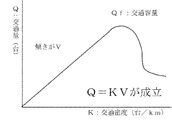•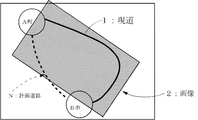•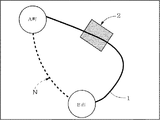•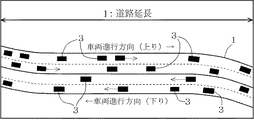•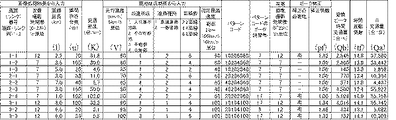•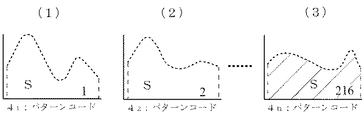•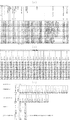•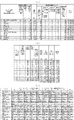•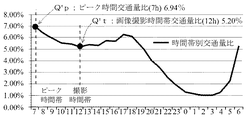## Abstract

PROBLEM TO BE SOLVED: To solve the following problems: in a traffic condition observation using a satellite image or the like, at least two images captured with a time lag are required for measuring vehicle speed, resulting in complicated image acquisition operation and cost increase.SOLUTION: A traffic estimation system acquires a single image (2) at one time point including a target current road (1) as a satellite image, an aerial photograph or Google (TM) map. The instantaneous number (n) of vehicles (3) existing on the current road in the image is measured. Traffic density (K) is calculated from the instantaneous number of vehicles and extension (l) of the current load. Image-processing traffic volume (Qk) is calculated from the traffic density and moving speed (V). A conversion peak time traffic volume (Qh) is calculated from a peak correction coefficient (pf) corresponding to pattern codes (41, 42, ...4n) to be obtained from the image-processing traffic volume and a location condition result of the current road. Daily traffic volume (Qa) is calculated from the conversion peak time traffic volume and a time variation coefficient (tf) corresponding to the pattern code of the current road.

## Description

The present invention relates to a traffic volume estimation system using a single image, and more particularly to a system using a single image obtained as a satellite image, aerial photograph or Google map.

When planning new road maintenance, it is a basic matter to grasp the current road, that is, the status of existing roads (highways and general roads) (traffic volume and speed of surrounding network, road structure, etc.) It is common to use traffic volume data stocked so far, such as automatic volume measuring devices and road traffic census.

In addition, when there is no stocked data, or when considering changes in the network or changes in the socio-economic situation, a new local traffic survey will be conducted as necessary. In consideration of the above, etc., the traffic volume and travel speed of each vehicle type are measured by investigators and measuring equipment, but there are also problems such as the possibility of human measurement mistakes, increased investigation costs, and ensuring the safety of investigators. Exists.

Currently, the applicant of the present application is considering overseas business development, but in many developing countries, stocked traffic volume data often do not exist. For this reason, it is indispensable to conduct local traffic surveys, and it seems that surveys utilizing local people are being conducted by companies (consultants) with overseas know-how. However, if there is a problem in local security, there may be restrictions on measurement points and measurement methods, and if the reliability of measurement data that is the basis of business profitability needs to be verified, it will be a major issue.

Therefore, this time, we proposed a traffic estimation system that uses a single image as a technology to replace the local traffic survey, which does not require a large-scale field survey in Japan as well as overseas, including narrow streets. It is also possible to verify the reliability of measurement data such as grasping traffic in a wide area and surveying local traffic.

Conventionally, in the case of measuring traffic volume using satellite images, multiple images of the target road are acquired with a time difference, and the traffic density is calculated from the number of vehicles appearing in these images and the road extension, The traffic volume is measured by multiplying this by the speed obtained from the moving distance of the vehicle between the images.

JP 2005-56186 A JP 2001-188986 A

Japanese Patent Laying-Open No. 2005-56186 (Patent Document 1) describes a method of capturing a plurality of images at a predetermined time interval (Δt) in paragraph “0014” and a method of measuring the number of vehicles from “0036” to “0039”. , Respectively.

Japanese Patent Laid-Open No. 2001-188986 (Patent Document 2) takes a satellite photograph several times in a short time of several seconds to several tens of seconds in the section “Means for Solving the Problems”. Then, the average mileage of a plurality of vehicles specified by comparing the images before and after is divided by this time to obtain the average mileage, and the result of dividing the average mileage by the average headway distance is the traffic volume. There is a statement to do so.

In the traffic condition observation system of Patent Document 1, the first image captured at the first time and the second image captured at the second time are necessary, and it is difficult to obtain both images. And an increase in cost is inevitable.

As in the case of Patent Document 1, the method of measuring the vehicle traveling speed and traffic volume on the road using the aerial photograph of Patent Document 2 is also used to take a plurality of satellite photographs at different times. The traffic volume is calculated using the displacement amount of the position of the same vehicle. Also in this case, it is inevitable that the work is difficult to obtain images by taking a plurality of satellite photographs and the cost is increased.

Therefore, an object of the present invention is to provide a traffic volume estimation system that can be performed with a single image and can be performed without labor and cost. In the following description, the case where the meanings such as “multiply” and “synergize” are expressed may be replaced by the symbol “×”, and the case where “divide” may be expressed by the symbol “÷”.

(Claim 1) A traffic estimation system using a single image according to the present invention obtains a single image at a certain point in time including a current road to be investigated as a satellite image, an aerial photograph or a Google map. To do. The instantaneous number of vehicles on the current road of the image is measured. The traffic density is calculated from the instantaneous number of vehicles and the road extension of the current road measured from the image or map. The image processing result traffic volume is calculated from the traffic density and the traveling speed. The converted peak time traffic volume is calculated from the image processing result traffic volume and the peak correction coefficient corresponding to the pattern code obtained from the local situation result of the current road. Then, the daily traffic volume is calculated from the converted peak hour traffic volume and the time variation coefficient corresponding to the pattern code of the current road.

The image is assumed to be a single image picked up by selecting a whole area or a representative section of the current road competing with the planned road, for example. The instantaneous number of vehicles on the current road shown in this image is measured. This measurement is performed by normal image processing using analysis software, or by visual observation by a person.

The extension of the current road where the vehicle is present is measured from the image or map data. The traffic density is calculated from the number of vehicles and the current road extension. As the traveling speed of the vehicle on the current road, the survey value collected by site inspection or the assumed value is adopted.

An image processing result traffic volume is calculated from the traffic density and the travel speed. The converted peak time traffic volume is calculated from the image processing result traffic volume and the peak correction coefficient corresponding to the pattern code obtained from the local situation of the current road. Then, the daily traffic volume is calculated from the converted peak hour traffic volume and the time variation coefficient corresponding to the pattern code of the current road. This peak correction is performed when the image shooting time zone is different from the peak time zone.

As shown in Fig. 1, between traffic volume Q (vehicles), traffic density K (vehicles / km), and travel speed V (km / h), the traffic volume is up to the traffic capacity. It is known that there is a relationship that increases in proportion to.

(Claim 2) The pattern code is obtained by extracting / aggregating survey item data for each survey section of each road type possessed by the road traffic census "roadside situation, road type, number of lanes, designated maximum speed" Alternatively, 216 road types may be newly classified, and the peak correction coefficient and the time variation coefficient may be provided for each classification.
In this way, by preparing various types of pattern codes, a pattern code of a road similar to the current road can be easily extracted and collated on a personal computer screen, and the peak correction coefficient and the The time variation coefficient can be easily obtained, and the traffic volume can be accurately estimated by applying the peak correction coefficient and the time variation coefficient.

(Claim 3) The peak correction coefficient is calculated by the ratio of peak hour traffic volume / image shooting time zone traffic volume ratio. The peak hour traffic volume ratio is the top 10 for 24 hour traffic for each pattern code. Extracting the data up to and extracting the traffic volume ratio of the peak hour traffic volume from the traffic volume ratio averaged for each time zone by dividing each hour traffic volume by the 24-hour traffic volume, Further, the image shooting time period traffic volume ratio may be obtained by extracting the traffic volume ratio of the image shooting time period.
In this way, as described below, the reliability and practicality of traffic estimation can be improved.

The peak hour traffic volume ratio, the image shooting time zone traffic volume ratio and the “traffic volume ratio” are used, for example, as shown in [Table 1] below, based on the I / II calculated by the traffic volume and the traffic volume ratio. This is because when I calculate and compare III / IV, I / II is influenced by the difference in number of units in the average value.

Therefore, by using the peak time traffic volume ratio and the image capturing time zone traffic volume ratio in this way, the peak correction coefficient can be calculated without being affected by the magnitude of the traffic volume.

The peak correction coefficient is a coefficient necessary for calculating the daily traffic volume, and it is as follows if it is explained from another viewpoint that the reliable daily traffic volume cannot be estimated unless this coefficient is multiplied.
Image processing result traffic volume x peak correction coefficient = conversion peak hour traffic volume conversion peak hour traffic volume x time variation coefficient = daily traffic time variation coefficient = day / night rate / daytime peak 12 hour ratio (% not shown) ,
Daily traffic = conversion peak hour traffic x day / night rate / daytime peak 12 hour ratio Day / night rate / daytime peak 12 hour ratio = 24 hour traffic / peak hour traffic
Daily traffic volume = Conversion peak hour traffic volume x 24 hour traffic volume / Peak hour traffic volume = 24 hour traffic volume = Daily traffic volume In other words, if the image processing result traffic volume is not multiplied by the peak correction factor, the conversion peak hour traffic volume will be As a result, daily traffic volume cannot be estimated.

(Claim 4) The time variation coefficient is calculated by day / night rate / daytime peak 12 hour ratio × 100,
The day / night rate is calculated by dividing the 24-hour traffic volume, which is the sum of the hourly traffic volumes from 7:00 to 7:00 the next day, by the 12-hour daytime traffic volume, which is the sum of the hourly traffic volumes from 7:00 to 19:00. thing,
The daytime peak 12 hour ratio is obtained by dividing the peak hour traffic volume which is the maximum hour traffic volume among each hour traffic volume by the daytime 12 hour traffic volume which is the total of each hour traffic volume from 7:00 to 19:00. It may be a thing.
In this way, as described in the previous paragraph “0022”, the time variation coefficient can be obtained, and the daily traffic volume can be estimated with high reliability.

(Claim 5) The time variation coefficient is obtained by calculating the 24-hour traffic volume that is the sum of the hourly traffic volumes from 7:00 to 7:00 the next time, and the peak hourly traffic volume that is the maximum hourly traffic volume of each hourly traffic volume. The value divided by may be multiplied by 100.
This produces the same effect as described in the preceding paragraph “0023”.

(Claim 6) The input conditions for calculating the daily traffic volume (Qa) are as follows:
(a) Input item of image processing result of the image (2) of the current road (1)
(a-1) Image shooting time zone (0-23 hour increments)
(a-2) Instantaneous units (n) (units)
(b) Input items for local status results
(b-1) Travel speed (V) (km / h)
(b-4) Number of lanes (selection)
(b-5) Designated maximum speed (selection)
The calculation result may be displayed on the input screen.
In this way, it is possible to know the estimated traffic volume of the current road on the input screen simply by satisfying the input items with the numerical values calculated on the desk, without conducting a local traffic volume survey.

(Claim 1) According to the traffic volume estimation system using an image according to the present invention, a single image at a certain point in time including a current road to be investigated is obtained as a satellite image, an aerial photograph or a Google map. Therefore, it can be applied to road planning in mountains, forests, and wetlands where it is difficult for people to enter, and it is possible to estimate traffic volume with high accuracy.

According to claim 2, a pattern code of a road similar to the current road can be easily extracted and collated on a personal computer screen, and the peak correction coefficient and the time variation coefficient can be easily obtained based on the pattern code. Therefore, the traffic volume can be accurately estimated by applying the peak correction coefficient and the time variation coefficient.

According to claim 3, the daily traffic volume can be calculated, and the practicality and reliability of the traffic volume estimation can be improved.

According to claims 4 and 5, the daily traffic volume can be calculated, and the practicality and reliability of the traffic volume estimation can be improved.

According to the sixth aspect, the estimated traffic volume of the current road can be known on the input screen.

It is a graph which shows the relationship between traffic volume Q (vehicle / h), traffic density K (vehicle / km), and traveling speed V (km / h). In the specific example of the traffic volume estimation system using the image concerning this invention, it is a conceptual diagram at the time of making the object of the image 2 into the full length of the present road 1. FIG. It is a conceptual diagram at the time of making it a part of the present road 1 similarly. This figure shows an example of a desktop calculation that calculates the traffic density K from the number of instantaneous vehicles n and the extension 1 of the current road 1. If the extension l is 2 km, the uphill is K = 12 vehicles / 2 km, 6 vehicles / km. Downhill is 4 cars / km with K = 8 cars / 2km. It is a figure which shows the state which gave data to this by giving an example of the input screen which analysis software displays with a personal computer. (1), (2) and (3) are examples extracted from 216 kinds of daily traffic pattern codes. (1) is No. 1, (2) is No. 2, (3) is Indicates No. 216. (a), (b), and (c) are materials explaining each index from the census sample data given as an example. The left end of (b) is continuous with the right end of (a). (c) is displayed below it, and shows how to calculate `` time variation coefficient '', `` 24-hour traffic volume '', `` daytime 12-hour traffic volume '', `` day / night rate '', and `` daytime peak 12-hour ratio ''. Yes. (a), (b), and (c) are sample demonstration diagrams for confirming the practicality of the pattern code. The “deviation rate (%) between the measured traffic volume and the 2010 traffic census traffic volume. ”Shows its practicality. This figure originally shows a single table, but because it is horizontally long, the fields of `` Pattern Code '' and `` Pattern Code Peak Time Zone '' are separated by a dashed line (a) and (b). Yes. (c) is the original data of traffic volume by time zone adopted in this system. An example of the pattern code is 10206060.

(Claim 1) A traffic volume estimation system utilizing a single image according to the present invention is a satellite image, an aerial photograph, or a single image 2 at a certain point in time including a current road 1 competing with a planned road N. Get as Google Maps. The instantaneous number n of vehicles 3 on the current road 1 in the image 2 is measured. The traffic density K is calculated from the instantaneous number n and the extension 1 of the current road 1. If this system is used, calculation after calculation of traffic density and selection of pattern codes 4 1, 4 2, ... 4 n can be automatically performed. The traveling speed V of the vehicle 3 on the current road 1 is measured. The traffic density K is multiplied by the traveling speed V to calculate the image processing result traffic volume Qk = K × V. Furthermore, when the image shooting time zone is different from the peak time zone, it corresponds to the pattern code 4 1 , 4 2 ,... 4 n obtained from the image processing result traffic volume Qk and the local situation result of the current road 1 The converted peak time traffic volume Qh = Qk × pf is calculated by multiplying by the peak correction coefficient pf. Then, calculating the pattern code 4 1 conversion peak time traffic Qh and Genmichi 1, 4 2, day from the time variation coefficient tf corresponding to ... 4 n traffic Qa.

As shown in Fig. 1, between traffic volume Q (vehicles / h), traffic density K (vehicles / km), and travel speed V (km / h), traffic volume Q reaches traffic capacity Qf, traffic density K There is a relationship that increases in proportion to the synergistic product of travel speed V.

The image is taken by selecting the entire area of the current road 1 competing with the planned road N as shown in FIG. 2, or selecting a representative section as shown in FIG. The instantaneous number n of vehicles 3 on the current road 1 shown in the image 2 is measured by normal image processing using analysis software, or by visual observation by a person.

In FIG. 4, the extension l of the current road 1 where the vehicle 3 exists is measured. For this, if there is a field measurement value, it is adopted, and if there is no field measurement value, it is measured from image 2 or map data. The traffic density K is calculated from the instantaneous number n of vehicles 3 and the extension l of the current road 1. As the traveling speed V of the vehicle 3 on the current road 1, a survey value collected by site inspection or an assumed value considering the topography is adopted.

In FIG. 5, the converted peak time traffic volume Qh is calculated by synergizing these traffic density K, travel speed V and peak correction coefficient pf. Then, the daily traffic volume Qa is calculated by multiplying the traffic volume Qh by the time variation coefficient tf.

(Claim 2) The pattern code 4 1, 4 2, ... 4 n is obtained from the survey item data for each survey section of each road type held by the road traffic census. 216 road types are newly classified from “number of lanes, designated maximum speed”, and the peak correction coefficient pf and time variation coefficient tf are provided for each of these classifications.
In this case, by providing a wide variety of pattern codes, matching and easily extract the pattern code 4 n-x of the road that is similar to the existing road 1 (where 0 <x <integer n) on computer screen can, this pattern based on the code 4 n-x prompts facilitate peak correction factor pf and time variation coefficient tf, it allows the estimation of the accurate traffic volume by application of the peak correction factor and time variation coefficient tf.

FIG. 6 shows three examples of 216 types of pattern codes that currently exist. This number of 216 includes four types of “roadside status”, two types of “road type”, three types of “number of lanes”, and “designated maximum speed” in the “input from local situation result” column of FIG. Nine types are multiplied. In practice, as shown in the “pattern code” column of FIG. 5, one pattern code is represented by an 8-digit number. The most significant 2 digits are 1 to 4 with the numbers 1 to 4 with “roadside situation” divided into 4 types, and the next 2 digits are 1 and 2 with 2 types of roads. Numbers including the 10's place, 3rd place's 1st digit shows numbers for each lane number 2, 4, 6 and the 3rd place of the last 3 digits are "designated maximum speed" of 20-100km / h In the range, the corresponding numerical value is shown in units of 10km / h.

FIG. 5 shows an example of an input screen displayed by the analysis software. When data is input to “input from image processing result” in the leftmost column, “traffic density (units / km)” K in the right adjacent column is calculated. . Next, an input is made in the “input from local situation result” field on the right side of the column, a similar waveform is selected from the road traffic census, and it is input in the “pattern code” on the right side. As a result, the necessity is determined in the lower left column of “Peak Correction” in the third column on the right side, and if necessary, the “correction coefficient” pf on the right is calculated, and the “Conversion Peak” in the right column is calculated. Time traffic volume (units / h) “Qh” is calculated. Further, based on this, a “time variation coefficient” tf is expressed, and based on this, “daily traffic volume (vehicles / day)” Qa in the rightmost column is calculated.

As an example, the pattern code 10206060 shown in FIG. 9 is the highest 10 with the roadside in a population-intensive area, the next 20 with the road type as a general road, the third with 6 lanes and 6 lanes, the lowest 060 indicates that the specified maximum speed is 60 km / h.

Here, x is an arbitrary integer that satisfies the condition of 0 <x <n. Since 216 types of pattern codes currently exist as described above, x can be any integer from 1 to 215. The peak time traffic ratio Q'p shown in the extracted pattern code 4 n-x is divided by the image capturing time period traffic ratio Q't, peak correction factor pf is calculated.

In this case, extraction of the pattern code 4 n-x of the road that is similar to the existing road 1 can be easily collated on the computer screen, the peak correction factor pf and time variation coefficient tf based on the pattern code 4 n-x It can be easily calculated, and the traffic volume can be accurately estimated by applying the peak correction coefficient pf and the time variation coefficient tf.

Figure 7 is divided into (a), (b), and (c), (a) and (b) are a single table, and (c) is appended below this single table. Thus, it is a material explaining each index from census sample data. The number of pattern codes in the leftmost column of (a), for example, 1 and 2, 3 to 6, is the same for 12 hours daytime traffic volume and 24 hours traffic volume. However, they are treated as individual data because they are not the same number on the item “Basic section number traffic volume survey”. For example, when a road section of 100 m is divided into a plurality of sections at connection points with other roads, for example, 100 m → No. 1: 10 m, No. 2: 50 m, No. 3: 40 m, and the like.

The method of obtaining five items from “time coefficient of variation” to “daytime peak 12 hour ratio” is described in the lower part of this document, taking the number of pattern codes as an example.
First, the “time variation coefficient” is 13.2 which is an average value obtained by dividing the total value 250.4 of the time variation coefficients of the pattern codes 1 to 19 by 19 of the number of pattern codes. There is a slight difference from the values in the table in the calculation after the decimal point.
As shown in the example of “Number of pattern codes” 1, “24-hour traffic volume” is 74,196 units in total from 7 am to 6 am the next day.
“Daytime 12-hour traffic” is 50,298 units in total from 7 am to 6 pm.
“Day / Night Rate” is 1.48, which is 74,196 units of 24-hour traffic divided by 50,298 units of 12-hour daytime.
The “daytime peak 12-hour ratio” is 11.3, which is the number of vehicles at 7,700 am, which seems to have the most traffic, divided by 50,298 vehicles at 12 hours in the daytime and multiplied by 100.

FIG. 8 is divided into (a), (b), and (c), but it is a diagram of sample demonstration on 12 lines to confirm the practicality of the pattern code, which is originally a single table. The original data adopted in this pattern code as the comparison target is as shown in the column entitled “Original data of hourly traffic volume adopted in this system” in the rightmost column. There is a “deviation rate (%)” between −20 and +25 between “daily traffic volume (vehicles / day) (Qa)” and “H22 census (vehicles / day) (Qs)” on each road. Although the average is -4.7%, it can be seen that there is no problem in practical use of the estimation.

This deviation rate (%) can be obtained by the following equation.
Deviation rate (%) = {daily traffic volume (units / day) Qa-H22 census (units / day) Qs} / H22 census (units / day) Qs
For example, in the case of sample number 1 (pattern code 10206080)
(Deviation rate) −6.0 (%) ≒ (69,722−74,196) / 74,196
It becomes.

(Claim 3) Peak correction factor pf is intended to calculate the peak time traffic ratio Q'p ÷ imaging time period traffic ratio Q't, the peak time traffic ratio Q'p is pattern code 4 1 , 4 2, ... 4 24 hours traffic every n extracts the data of the top 10, from the averaged traffic ratios each time traffic in each time zone is divided by the 24-hour traffic The traffic volume ratio in the time zone of the peak hour traffic volume Qp may be extracted, and the image traffic time zone traffic volume ratio Q't may be the traffic volume ratio in the shooting time zone of the image 2 extracted.
In this case, the daily traffic volume Qa can be calculated, and the practicality and reliability of the traffic volume estimation can be improved.

(Claim 4) The time variation coefficient tf is calculated by the day / night rate rd ÷ daytime peak 12 hour ratio rh × 100, and this day / night rate rd is the sum of the hourly traffic volume from 7:00 to 7:00 the following day. A certain 24-hour traffic volume divided by the 12-hour daytime traffic volume that is the sum of each hourly traffic volume from 7:00 to 19:00, and the daytime peak 12-hour ratio rh is the maximum hourly traffic volume. The peak hour traffic volume Qp, which is the traffic volume, may be divided by the daytime 12 hour traffic volume, which is the total of the hourly traffic volumes from 7:00 to 19:00.
As can be seen with reference to FIG. 7 given as an example, the “time variation coefficient” is 13.2 which is an average value obtained by dividing the total value 250.4 of the time variation coefficients of the pattern codes 1 to 19 by 19 of the number of pattern codes. . There is a slight difference from the values in the table in the calculation after the decimal point.
In this case, the daily traffic volume Qa can be calculated, and the practicality and reliability of the traffic volume estimation can be improved.

(Claim 5) The time fluctuation coefficient tf is the 24-hour traffic volume that is the sum of each hourly traffic volume from 7:00 to 7:00 the next time, and the peak hourly traffic volume that is the maximum hourly traffic volume of each hourly traffic volume. The value divided by Qp may be multiplied by 100.
In this case, the result is the same as that in the previous paragraph 0045, but the coefficient can be easily calculated.

(Claim 6) Daily traffic volume Qa calculation input conditions are as follows:
(a) Input item of image processing result of image 2 of current road 1
(a-1) Image shooting time zone (1 hour unit at 0-23 o'clock)
(a-2) Instantaneous units (n) (units)
(b) Input items for local status results
(b-1) Travel speed (V) (km / h)
(b-4) Number of lanes (selection)
(b-5) Designated maximum speed (selection)
The calculation result may be displayed on the input screen.
In this case, it is possible to know the estimated traffic volume of the current road on the input screen only by satisfying the input items with the numerical values calculated on the desk without conducting a local traffic volume survey.

3 Vehicle
4 1, 4 2, ... 4 n pattern code
n Instantaneous units
K traffic density
V Travel speed
Q Traffic volume
Qk Image processing result traffic volume
pf Peak correction factor
Qh conversion peak hour traffic
tf Coefficient of time variation
Qa Daily traffic
Qd Daytime traffic
Q'p Peak hour traffic ratio
Qp Peak hour traffic
Q't image shooting time traffic ratio
Qs Traffic volume by time
rd day / night rate
rh daytime peak 12 hour ratio

## Claims (6)

1. Obtain a single image (2) at one point including the current road (1) to be surveyed as a satellite image, aerial photograph or Google map,
Measure the instantaneous number (n) of vehicles (3) on the current road (1) in the image (2),
Calculate the traffic density (K = n / l) from the road extension (l) of the current road (1) measured from the instantaneous number (n) and the image (2) or map,
Calculate the image processing result traffic volume (Qk = K × V) from the traffic density (K) and travel speed (V),
From the image processing result traffic volume (Qk) and the peak correction coefficient (pf) corresponding to the pattern code (4 1 , 4 2 ,... 4 n ) obtained from the local situation result of the current road (1), the converted peak time traffic Calculate the quantity (Qh = Qk x pf)
Daily traffic volume (Qa = Qh × tf) from the converted peak time traffic volume (Qh) and the time variation coefficient (tf) corresponding to the pattern code (4 1 , 4 2 ,... 4 n ) of the current road (1) ) Traffic volume estimation system using a single image.
2. The pattern code (4 1 , 4 2 ,... 4 n ) is obtained by extracting and aggregating survey item data for each survey section of each road type held by the road traffic census. 2. The traffic volume estimation system utilizing a single image according to claim 1, wherein 216 road types are newly classified from "number, designated maximum speed", and each peak has a value of the peak correction coefficient and the time variation coefficient for each classification. .
3. The peak correction coefficient (pf) is calculated by the peak time traffic volume ratio (Q'p) ÷ image shooting time zone traffic volume ratio (Q't),
The peak hourly traffic volume ratio (Q'p) is obtained by extracting the data for the top 10 of the 24-hour traffic volume for each of the pattern codes (4 1 , 4 2 ,... 4 n ). The traffic volume ratio for the peak hour traffic volume is extracted from the traffic volume ratio divided by the 24-hour traffic volume and averaged for each time zone.
The traffic using the single image according to claim 1 or 2, wherein the traffic ratio (Q't) of the image photographing time period is obtained by extracting the traffic volume ratio of the photographing time period of the image (2). Quantity estimation system.
4. The time variation coefficient (tf) is calculated by the day / night rate (rd) ÷ daytime peak 12 hour ratio (rh) × 100,
The day / night rate (rd) is the 24-hour traffic volume that is the sum of the hourly traffic volumes from 7:00 to 7:00 the following day, and the daytime 12-hour traffic volume that is the sum of the hourly traffic volumes from 7:00 to 19:00. Divided by
The daytime peak 12 hour ratio (rh) is the peak hourly traffic volume (Qp), which is the maximum hourly traffic volume among each hourly traffic volume, and the daytime 12 hours which is the total of each hourly traffic volume from 7:00 to 19:00. The traffic volume estimation system using a single image according to claim 1, 2 or 3, wherein the traffic volume is divided by the hourly traffic volume.
5.   The time variation coefficient (tf) is calculated as follows: 24-hour traffic volume, which is the total of each hourly traffic volume from 7 o'clock to 7 o'clock the next time, and the peak hourly traffic volume (Qp 4. The traffic volume estimation system using a single image according to claim 1, 2 or 3, wherein the value divided by 100 is multiplied by 100.
6. The input conditions for calculating the daily traffic volume (Qa) are as follows:
(a) Input item of image processing result of the image (2) of the current road (1)
(a-1) Image shooting time zone (1 hour unit at 0-23 o'clock)
(a-2) Instantaneous units (n) (units)
(b) Input items for local status results
(b-1) Travel speed (V) (km / h)
(b-4) Number of lanes (selection)
(b-5) Designated maximum speed (selection)
The traffic volume estimation system using a single image according to claim 1, wherein the calculation result is displayed on an input screen.

JP2012035956A 2012-02-22 2012-02-22 Traffic estimation system using single image Pending JP2013171491A (en)

## Priority Applications (1)

Application Number Priority Date Filing Date Title
JP2012035956A JP2013171491A (en) 2012-02-22 2012-02-22 Traffic estimation system using single image

## Applications Claiming Priority (1)

Application Number Priority Date Filing Date Title
JP2012035956A JP2013171491A (en) 2012-02-22 2012-02-22 Traffic estimation system using single image

## Publications (1)

Publication Number Publication Date
JP2013171491A true JP2013171491A (en) 2013-09-02

# Family

## Family Applications (1)

Application Number Title Priority Date Filing Date
JP2012035956A Pending JP2013171491A (en) 2012-02-22 2012-02-22 Traffic estimation system using single image

## Country Status (1)

JP (1) JP2013171491A (en)

## Cited By (2)

* Cited by examiner, † Cited by third party
Publication number Priority date Publication date Assignee Title
WO2015134453A1 (en) * 2014-03-03 2015-09-11 Inrix Inc. Estimating traveler volume by evaluating aerial images
CN106097726A (en) * 2016-08-23 2016-11-09 苏州科达科技股份有限公司 The detection determination in region, traffic information detection method and device

## Cited By (4)

* Cited by examiner, † Cited by third party
Publication number Priority date Publication date Assignee Title
WO2015134453A1 (en) * 2014-03-03 2015-09-11 Inrix Inc. Estimating traveler volume by evaluating aerial images
EP3114662A4 (en) * 2014-03-03 2017-12-13 Inrix, Inc. Traffic flow rates
US10319232B2 (en) 2014-03-03 2019-06-11 Inrix Inc. Traffic flow rates
CN106097726A (en) * 2016-08-23 2016-11-09 苏州科达科技股份有限公司 The detection determination in region, traffic information detection method and device

## Similar Documents

Publication Publication Date Title
Ji et al. Characterizing urban sprawl using multi-stage remote sensing images and landscape metrics
Renschler et al. Soil erosion assessment tools from point to regional scales—the role of geomorphologists in land management research and implementation
Mantovani et al. Remote sensing techniques for landslide studies and hazard zonation in Europe
Lin et al. Assessing debris-flow hazard in a watershed in Taiwan
Nuth et al. Decadal changes from a multi-temporal glacier inventory of Svalbard
Yigitcanlar et al. A GIS‐based land use and public transport accessibility indexing model
Bhatta et al. Quantifying the degree-of-freedom, degree-of-sprawl, and degree-of-goodness of urban growth from remote sensing data
Yigitcanlar et al. Developing a sustainability assessment model: The sustainable infrastructure, land-use, environment and transport model
Williams et al. Synthesis of transportation applications of mobile LiDAR
Wang et al. Visual exploration of sparse traffic trajectory data
Bauer et al. Estimating and mapping impervious surface area by regression analysis of Landsat imagery
Schneider et al. Pilot model for estimating pedestrian intersection crossing volumes
Ioannidis et al. Towards a strategy for control of suburban informal buildings through automatic change detection
Cheng et al. Measuring urban job accessibility with distance decay, competition and diversity
Salzmann et al. Assessment of the hazard potential of ice avalanches using remote sensing and GIS‐modelling
Goetz Towards generating highly detailed 3D CityGML models from OpenStreetMap
Gumma et al. Mapping irrigated areas of Ghana using fusion of 30 m and 250 m resolution remote-sensing data
Fiedler et al. Evaluation of noise pollution in urban traffic hubs—Noise maps and measurements
Yagoub Monitoring of urban growth of a desert city through remote sensing: Al-Ain, UAE, between 1976 and 2000
Kasemsuppakorn et al. A pedestrian network construction algorithm based on multiple GPS traces
Romano et al. Multi-criteria decision analysis for land suitability mapping in a rural area of Southern Italy
White et al. Forest roads mapped using LiDAR in steep forested terrain
Griswold et al. Pilot models for estimating bicycle intersection volumes
Estoque et al. Intensity and spatial pattern of urban land changes in the megacities of Southeast Asia
Liang et al. Assessing urban environmental quality change of Indianapolis, United States, by the remote sensing and GIS integration